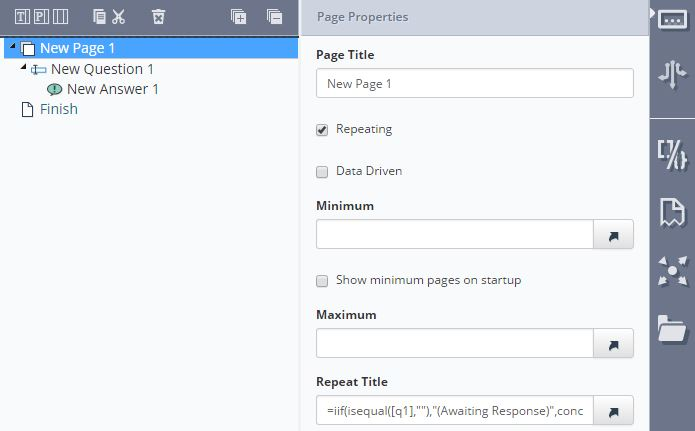# Dynamic repeating page names

Repeating Sections can have fixed or dynamic page for example if a repeating page is to collect information about students, the page name could include the Student’s name so you can quickly navigate to a particular student in Produce.

To achieve this use a formula in the repeating page title like the one below that concatenates the word ‘StudentData’ with the response to question 1 (note: formulas must start with an ‘=’ symbol).

``````=concat("Student Data (",[q1],")")
``````

The above formula may look incomplete until the Q1 has a value this can be overcome by using an iif formula:

``````=iif(isequal([q1],""),"(Awaiting Response)",concat("Student Data (",[q1],")"))
``````